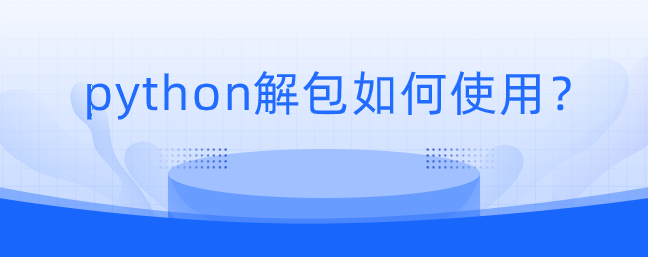# python解包如何使用？1、使用说明

（1）解包的意义就是将传递给函数的一个列表，元组，字典，拆分成独立的多个元素然后赋值给函数中的形参变量。

（2）解包字典有两种解法，一种用*解的只有key，一种用**解的有key、value。但是这个方法**只能在函数定义中使用。

2、*方法

（1）列表解包

```a, b, c = [1, 2, 3]
print(a, b, c)
# 1 2 3```

（2）元祖解包

```a, b, c = (1, 2, 3)
print(a, b, c)
# 1 2 3```

（3）字符串解包

```a, b, c = "abc"
print(a, b, c)
# a b c```

（4）字典解包

```a, b, c = {"a": 1, "b": 2, "c": 3}
print(a, b, c)
# a b c```

```def test():
return 1, 2, 3

a, b, c = test()
print(a, b, c)      # 1 2 3```

3、**方法函数解包

```def test():
return 1, 2, 3

a, *b = test()
print(a, b)     # 1 [2, 3]```

Python开发开发项目